Next: Resistive Impedance Up: Alternating Current Circuits Previous: RCL Circuit

# Sinusoidal Sources and Complex Impedance

We now consider current and voltage sources with time average values of zero. We will use periodic signals but the observation time could well be less than one period. Periodic signals are also useful in the sense that arbitrary signals can usually be expanded in terms of a Fourier series of periodic signals. Lets start with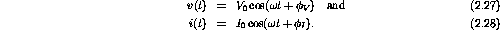Notice that I have now switched to lowercase symbols. Lowercase is generally used for AC quantities while uppercase is reserved for DC values.

Now is the time to get into complex notation since it will make our discussion easier and is encountered often in electronics. The above voltage and current signals can be written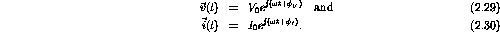To be cleaver we will define one EMF in the circuit to have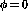. In other words, we will pick t=0 to be at the peak of one signal. The vector notation is used to remind us that complex numbers can be considered as vectors in the complex plane. Although not so common in physics, in electronics we refer to these vectors as phasors. Hence you should now review complex notation.

The presence of sinusoidal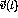or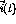in circuits will result in an inhomogeneous differential equation with a time-dependent source term. The solution will contain sinusoidal terms with the source frequency.

The extension of Ohm's law to AC circuits can be written as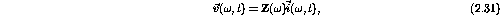whereis the source frequency.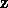is a generalized resistance referred to as the impedance.

We can cancel out the common time dependent factors to obtain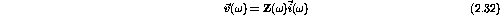and hence you see the power of the complex notation. For a physically quantity we take the amplitude of the real signal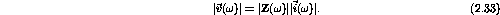We will now examine each circuit element in turn with a voltage source to deduce its impedance.Next: Resistive Impedance Up: Alternating Current Circuits Previous: RCL Circuit

Doug Gingrich
Tue Jul 13 16:55:15 EDT 1999